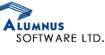# Placement Papers - AlumnusAlumnus Placement Paper Pattern
Posted by :
Janardhan
(13)
Alumnus Placement Paper Pattern

Alumnus Technical - Java

Sample Test paper

1. Find the locus of the point whose sum of distance from 2 fixed points is constant.

2. [-infinity to +infinity] integration (exp(-x*x))

3. The product of any 3 consecutive nos is always divisible by _ ? options[12/6/10/24]

4  int i=0,j=50
while (i<j)
{
if(<some condtn>)
{
<body of the loop>
i++
}
elseif(<some condtn>)
{  <body of the loop>
j--
}
else(<some condtn>)
{<body of the loop>
j--
}
}

How many times the body of the loops going to be executed? options [unknown/25/50/depends on the given condition]

5  How can you include a library code written in C++ in a source code written in C (Options are there)

Ans. Some cross-linked platform (compiler) is required for this.

6  int a,i
for(i=0;i<20;i++)
{
a[i]=i;
}
for(i=0;i<20;i++)
{
a[i]=a[20-i]
}
What is the final content of the program.

7   Which data structure should be used for searching an element in an array in constant time in case of
average case?  Ans. Hash table (not sure)

8   One question on ISO OSI model. easy one (not remembered)

9   Another question about. a protocol Ans. Token/Ring

10  If we want to connect two systems to form a network which leyer(OSI) is of most interest to us?

Ans. Physical layer

Alumnus Technical - C & C++

Each question had 5 options. There were 25 question in all and all were Objective Type.

1   When compiled from command line what are linking options?

2   You have written a code in C++, and u have to use a C library , what would u do?

Ans: write extern "c" in header files)
3   Fiber optic backbone is in which OSI layer?

4   void main()
{
int x=1;
int y=1;
int i;
for(i=2;i<=100;i++)
{
x=x+i;y=y*(i+1)/(i-1);
}
What are the values of x & y?

5  If we carry out operation (-3)+(-6),then which of the what will be the value of carry and sign flag?

6  void abc(int a[])
{
int k=0;int j=50;
while(k<j)
{
if(a[i]>a[j])
k++;
else
j--;
}
How many times the loop will occur?

7   Integrate e^(x^-2)dx , with limits -infinity to +infinity?What is the final value?

8   Let p be a 16 bit number.The 2,s complement of p will be represented by?

9   void main()
{
int a[]={5,4,3,2,1};
int x,y;
int *p=&a;
*p++;
x=++*p;
y=*(p++);
printf("%d %d",x,y),
}
What will be the value of x and y?

10   Let there be a set of 3 numbers. Then number of groups possible?

11   A question on some technique used in DA-converter?

12   Which data structure to use for fastest search?

13   A binary tree contains 1024 elements.What is maximum number of comparisons needed to search an element?

14   s=1-1/4+1/16-1/32...... What is the value of S?(Ans = 0.8 Hint Its a GP)

15   A C++ class has multiple references to base class.Then some options were given?

16   for(i=0;i<20;i++)
{
a[i]=i;

}
for(i=0;i<20;i++)
{
a[i]=a[19-i];
}
What is final value of array a? Options were there.
17  In Java can a variable be initilised inside a loop?

18  Two dices are thrown. What is the probality that the the number on the first dice is greater or equal to number on the second dice?